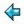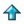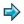### set.m

```||definition of finite sets as an abstract data type, say
||      %include <ex/set>
||to include this in one of your own scripts.

abstype set *
with  makeset :: [*]->set *             ||converts list to set
enum :: set *->[*]                ||converts set to list
empty :: set *                    ||empty set
mem :: set *->*->bool             ||does set contain element?
pincludes, includes
:: set *->set *->bool             ||(proper) set inclusion
union :: [set *]->set *           ||union of a list of sets
intersect :: [set *]->set *       ||intersection of a list of sets
setdiff :: set *->set *->set *    ||set difference of two sets
union2 :: set *->set *->set *     ||union of two sets
intersect2 :: set *->set *->set * ||intersection of two sets
add1 :: *->set *->set *           ||add a single element to a set
sub1 :: *->set *->set *           ||remove an element from a set (if present)
pick :: set *->*                  ||pick some element from a set
rest :: set *->set *              ||remainder of set, without element got by pick
showset
:: (*->[char])->set *->[char]     ||make sets printable

set * == [*]        ||a set is represented as a list without duplicates
makeset = uniq.sort ||for efficiency the lists are kept sorted
enum = id
empty = []
mem (a:x) b = a=b \/ a<b & mem x b
mem [] b = False
includes x y = (setdiff y x = [])
pincludes x y = x~=y & (setdiff y x = [])
union2 x y = uniq(merge x y)
union = foldr union2 empty
setdiff (a:x) (b:y) = a:setdiff x (b:y), if a<b
= setdiff (a:x) y,   if a>b
= setdiff x y,       otherwise
setdiff x y = x
intersect2 (a:x) (b:y) = intersect2 x (b:y), if a<b
= intersect2 (a:x) y, if a>b
= a : intersect2 x y, otherwise
intersect2 x y = []
intersect = foldl1 intersect2
add1 a (b:x) = a:b:x,      if a<b
= b:x,        if a=b
sub1 a (b:x) = b:x,        if a<b
= x,          if a=b
= b:sub1 a x, otherwise
sub1 a [] = []
pick (a:x) = a
pick [] = error "pick empty"
rest (a:x) = x
rest [] = error "pick empty"
showset f [] = "{}"
showset f (a:x) = "{"++f a++concat(map g x)++"}"
where
g a = ',':f a

%export -uniq    ||export all except uniq

uniq::[*]->[*]    ||removes adjacent duplicates from a list
uniq (a:b:x) = uniq (a:x),   if a=b
= a:uniq (b:x), otherwise
uniq x = x
```